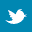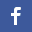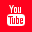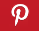Completely Solved C, C++ Programs Assignment.Quick Search Database Videos Tutorials Ebooks Forums FAQ Aboutus Household Industrial Manufacturing Service Shopping Transportation### C Programs

 Filed Under:

C PROGRAMMING
Inventor:          Denis Ritchie

Compiler:         TURBO C++ 5     BORLAND C++ 4.5       DEV C++

Ref.Books:       Let Us C by Yashvant Kanetkar
C by Denis Ritchie
Balaguruswami

Basic C Program:

# 3.    write a program in C to find the area of triangle .

4.    Write a program in C to find the area of sphere
5.    Write a program in C to find the circumference of circle

# 6.    Write a program in C to find the area of circle using input value

7.    Write a program in C to find the area of triangle using input value
8.    Write a program in C to find the area of sphere using input value

# 9.    Write a program in C to find the circumference of circle using input value

10.  Write a program in C to read and print an given character
11.  Write a program in C to print the ascii value of given character

# 12.  Write a program in C to read and print the given integer

13.  Write a program in C to read and print the given float

# 18.  Write a program in C to Arithmetic operations of two integer numbers .Print these results in separate lines

19.  Write a program in C to Arithmetic operations of two long numbers . Print these results in separate      lines

# 24.  Write a program in C to read float value and print integer equivalent

25.  Write a program in C to read int value and print float equivalent

While Loop:
1.    Write a program in C to print the numbers 1 to 100
2.    Write a program in C to sum of first 100 numbers
3.    Write a program in C to sum of first N numbers
4.    Write a program in C to sum of M to N numbers
5.    Write a program in C to find the average of 1 to 100

# 10.  Write a program in C to display the even or odd numbers between to ranges

## 11.  Write a program in C to display the leap years between 2000 to 3000

12.  Write a program in C to display the leap years of given range
13.  Write a program in C to print the numbers between two ranges

# 14.  Write a program in C to print the number until -1000 is given a input

15.  Write a program in C to print the positive , negative and zero until -1000 is given a input

# 17.  Write a program in C to sum of the positive, negative numbers until -1000 is given a input

18.  Write a program in C to mean of the positive , negative numbers until -1000 is given a input

# 23.  Write a program in C to find smallest of three numbers using ternary operator

24.  Write a program in C to find biggest of ten numbers
25.  Write a program in C to find smallest of ten numbers
26.  Write a program in C to find biggest of N numbers

# 29.  Write a program in C to read and display of N numbers using array

30.  Write a program in C to find mean of ten numbers using array
31.  Write a program in C to find mean of N numbers using array

# 40.  Write a program in C to find the second biggest and smallest of N numbers using array

41.  Write a program in C to find the positions of second biggest and smallest of N numbers using array

If…Else:

# 11.  Write a program in C for given mark contain which grade

12.  Write a program in C to find biggest of two given numbers
13.  Write a program in C to find smallest of two given numbers
14.  Write a program in C to find biggest of three given numbers
15.  Write a program in C to find smallest of three given numbers

# 18.  Write a program in C to display the name of the day in a week. Here given input range is 1 to 7.

19.  Write a program in C to find an biggest of four given numbers.
20.  Write a program in C to find an smallest of four given numbers

Switch Case:

# 4.    Write a program in C to display the given character vowel or not

Dynamic Memory Allocation:

# 2.    Write a program in C to sum of two integer numbers

3.    Write a program in C to larger of two integer numbers

# 6.    Write a program in C to read and display text using dynamic allocation

7.    Write a program in C to read N characters

# 9.    Write a program in C to merge two arrays

10.  Write a program in C to read and display biodata using structures

# 14.  Write a program in C to read and display integer array using functions

15.  Write a program in C to read and display biodata using functions

# 18.  Write a program in C to print the sum of two 3 x 3 Matrix Write a program in C to print the product of two 3 x 3 Matrix

19.  Write a program in C to read and display a N x N Matrix
20.  Write a program in C to transpose the N x N Matrix

# 21.  Write a program in C to print the sum of two N x N Matrix

22.  Write a program in C to print the product of two N x N Matrix
23.  Write a program in C to read and display a M x N Matrix
24.  Write a program in C to transpose of M x N Matrix
25.  Write a program in C to print the sum of two M x N Matrix
26.  Write a program in C to print the product of two M x N Matrix

Perfect Number:
1.    Write a c program to check given number is perfect number or not.
2.    Write a C program to print the perfect number between 1to 1000.

Armstrong Number:
1.    Write a c program to check given number is Armstrong number or not.
2.    Write a C program to print the Armstrong number between 1to 1000.

Prime Number:
1.    Write a c program to check given number is prime number or not.
2.    Write a C program to print the prime number between 1to 1000.
3.    Write a c program to find out prime factor of given number (Using While Loop).
4.    Write a c program to find out prime factor of given number (With Recursion).
5.    Write a c program to find out prime factor of given number (Without Recursion).
6.    Write a c program to find Gold batch number (gold batch conjecture).
7.    Write a c program to find Twin prime number up to a given number.

Strong Number:
1.        Write a c program to check given number is strong number or not.
2.        Write a C program to print the strong number between 1to 1000

Triangle:
1.        Write a c program to print Pascal’s triangle.
2.        Write a c program for Floyd’s triangle.

Pattern:

LCM, HCF, GCD:

Reverse:
1.    Write a c program to reverse a number.
2.    Write a c program to reverse a string.

Palindrome:
1.    Write a C program whether a given number is palindrome or not.
2.    Write a C program whether a given String is palindrome or not.

Swapping:
1.    1.Write a c program to swapping two numbers (With using third variable).
2.    Write a c program to swapping two numbers (Without using third variable).
3.    Write a c program for swapping of two arrays.
4.    Write a c program for swapping of two strings.
Conversion:
1.    Write a c program to convert a number from any base to any base.
2.    Write a c program to convert decimal number to binary number.
3.    Write a c program to convert decimal number to octal number.
4.    Write a c program to convert decimal number to hexadecimal number.
5.    Write a c program to convert octal number to binary number.
6.    Write a c program to convert octal number to decimal number.
7.    Write a c program to convert hexadecimal number to binary number.
8.    Write a c program to convert binary number to octal number.
9.    Write a c program to convert binary number to decimal number.
10.  Write a c program to convert binary number to hexadecimal number.

11.  C program fractional binary conversion from decimal.
12.  C program for fractional decimal to binary fraction conversion.
13.  C program to convert decimal number to roman.
14.  C program to convert roman number to decimal number.
15.  C program to convert each digits of a number in words.
16.  C program to convert currency or number in word.
17.  C program for unit conversion.
18.  Write a c program to convert the string from lower case to upper case.
19.  Write a c program to convert the string from upper case to lower case.

Series:
1.    Write a c program to find out the sum of series 1 + 2 + ….  + n.
2.    Write a c program to find out the sum of series 1^2 + 2^2 + …. + n^2.
3.    Write a c program to find out the sum of series 1^3 + 2^3 + …. + n^3.
4.    Write a c program to find out the sum of given A.P.
5.    Write a c program to find out the sum of given G.P.
6.    Write a c program to find out the sum of series 1 + 2 + 4 + 8 … to infinity.

Recursion:
1.    Write a c program to reverse any number using recursion.
2.    Write a c program to find out sum digits of a number using recursion.
3.    Write a c program to find factorial of a number using recursion.
4.    Write a c program to find power of a number using function recursion.
5.    Write a c program to find GCD of a two numbers using recursion.

Array:
a)      Matrix & Determinant

# 3.        Write a program in C to print the sum of two 3 x 3 Matrix Write a program in C to print the product of two 3 x 3 Matrix

4.        Write a program in C to read and display a N x N Matrix
5.        Write a program in C to transpose the N x N Matrix

# 6.        Write a program in C to print the sum of two N x N Matrix

7.        Write a program in C to print the product of two N x N Matrix
8.        Write a program in C to read and display a M x N Matrix
9.        Write a program in C to transpose of M x N Matrix
10.     Write a program in C to print the sum of two M x N Matrix
11.     Write a program in C to print the product of two M x N Matrix
12.     Write a program in c to print determinant of N x N matrix
13.     Write a c program for subtraction of two matrices
14.     Write a c program for multiplication of two matrices.
15.     Write a c program to find out sum of diagonal element of a matrix.
16.     Write a c program to find out transport of a matrix.

b) General
1.    Write a c program for insert an element at desired position in an array.
2.    Write a c program to find out second smallest element of an unsorted array.
3.    Write a c program to find out largest element of an array.
4.    Write a c program for delete an element at desired position in an array.
5.    Write a c program which deletes the duplicate element of an array.
6.    Write a c program to find out second largest element of an unsorted array.

String:

File:

Bitwise Operator:

Sorting:
1.    Write a c program for bubble sort.
2.    Write a c program for insertion sort.
3.    Write a c program for selection sort.
4.    Write a c program for quick sort.
5.    Write a c program for heap sort.
6.    Write a c program for shell sort.
7.    Write a c program for radix sort.

Searching:
1.    Write a c program for linear search.
2.    Write a c program for binary search.
3.    Write a c program for binary search using recursion.

Largest Element:
1.    Write a c program to find factorial of 100 or very large numbers
2.    Write a c program to multiply the two very large number (larger than long int)
3.    Write a c program for division of large number (larger than long int)
4.    Write a c program for modular division of large number.
5.    Write a c program for power of large numbers.

Numerical Analysis:

Miscellaneous:
1.    Write a c program to find the size of int without using sizeof operator.
2.    Write a c program to find the size of double without using sizeof operator.
3.    Write a c program to find the size of structure without using sizeof operator.
4.    Write a c program to find the size of union without using sizeof operator.